# Introduction to the MachineShop Package

### Package Version 1.2.0

University of Iowa
brian-j-smith@uiowa.edu

# 1 Overview

## 1.1 Description

MachineShop is a meta-package for statistical and machine learning with a unified interface for model fitting, prediction, performance assessment, and presentation of results. Support is provided for predictive modeling of numerical, categorical, and censored time-to-event outcomes and for resample (bootstrap, cross-validation, and split training-test sets) estimation of model performance. This vignette introduces the package interface with a survival data analysis example, followed by supported methods of variable specification; applications to other response variable types; available performance metrics, resampling techniques, and graphical and tabular summaries; and modeling strategies.

## 1.2 Features

• Unified and concise interface for model fitting, prediction, and performance assessment.
• Current support for 49 established models from 25 R packages.
• Ensemble modeling with stacked regression and super learners.
• Modeling of response variables types: binary factors, multi-class nominal and ordinal factors, numeric vectors and matrices, and censored time-to-event survival.
• Model specification with traditional formulas and with flexible pre-processing recipes.
• Resample estimation of predictive performance, including cross-validation, bootstrap resampling, and split training-test set validation.
• Parallel execution of resampling algorithms.
• Choices of performance metrics: accuracy, areas under ROC and precision recall curves, Brier score, coefficient of determination (R2), concordance index, cross entropy, F score, Gini coefficient, unweighted and weighted Cohen’s kappa, mean absolute error, mean squared error, mean squared log error, positive and negative predictive values, precision and recall, and sensitivity and specificity.
• Graphical and tabular performance summaries: calibration curves, confusion matrices, partial dependence plots, performance curves, lift curves, and variable importance.
• Model tuning over automatically generated grids of parameter values and randomly sampled grid points.
• Model selection and comparisons for any combination of models and model parameter values.
• User-definable models and performance metrics.

# 2 Melanoma Example

The package is illustrated in the following sections with an overall survival analysis example in which the response variable is a time to event outcome. Since survival outcomes are a combination of numerical (time to event) and categorical (event) variables, package features for both variable types will be utilized in the example. Outcomes other than survival, including nominal and ordinal factors as well as numeric vectors and matrices, are supported by MachineShop and will be discussed.

Survival analysis is performed with the Melanoma dataset from the MASS package (Andersen et al. 1993). This dataset provides survival time, in days, from disease treatment to (1) death from disease, (2) alive at end of study, or (3) death from other causes for 205 Denmark patients with malignant melanomas. Also provided are potential predictors of the survival outcomes. The analysis begins by loading required packages MachineShop, survival, and MASS as well as magrittr (Bache and Wickham 2014) for its pipe (%>%) operator to simplify some of the code syntax. For the analysis, a binary overall survival outcome is created by combining the two death categories (1 and 3) into one. The dataset is then split into a training set on which a survival model will be fit and a test set on which predictions will be made. A global formula surv_fo is defined to relate the predictors on the right hand side to the overall survival outcome on the left and will be used in all of the survival models in this vignette.

## Analysis libraries
library(MachineShop)
library(survival)
library(MASS)
library(magrittr)

## Malignant melanoma analysis dataset
surv_df <- within(Melanoma, status <- as.numeric(status != 2))
Table 1. Variable summaries for the Melanoma survival analysis example.
Characteristic Value
Number of subjects 205
time
Median (Range) 2005 (10, 5565)
status
0 = Alive 134 (65.37%)
sex
1 = Male 79 (38.54%)
0 = Female 126 (61.46%)
age
Median (Range) 54 (4, 95)
year
Median (Range) 1970 (1962, 1977)
thickness
Median (Range) 1.94 (0.1, 17.42)
ulcer
1 = Presence 90 (43.9%)
0 = Absence 115 (56.1%)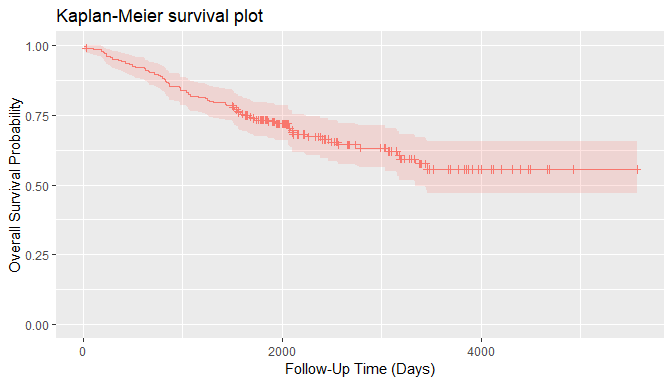## Training and test sets
set.seed(123)
train_indices <- sample(nrow(surv_df), nrow(surv_df) * 2 / 3)
surv_train <- surv_df[train_indices, ]
surv_test <- surv_df[-train_indices, ]

## Global formula for the analysis
surv_fo <- Surv(time, status) ~ sex + age + year + thickness + ulcer

# 3 Model Fitting and Prediction

Model fitting requires user specification of an available model. A named list of MachineShop models can be obtained interactively with the modelinfo function, and includes a descriptive "label", the source "packages" on which the models depend, supported response variable "types", and "arguments" that can be specified in calls to the model functions. Note that in order to use a model the source packages must be installed with the install.packages or equivalent function, but need not be loaded with the library function. Function modelinfo can be called with one or more model functions, function names, function calls, or observed response variables; and will return information on all models matching the calling arguments.

## All available models
modelinfo() %>% names
#>   "BARTMachineModel"    "BlackBoostModel"     "C50Model"
#>   "CForestModel"        "CoxModel"            "CoxStepAICModel"
#>  "EarthModel"          "FDAModel"            "GAMBoostModel"
#>  "GBMModel"            "GLMBoostModel"       "GLMModel"
#>  "GLMStepAICModel"     "GLMNetModel"         "KNNModel"
#>  "LARSModel"           "LDAModel"            "LMModel"
#>  "MDAModel"            "NaiveBayesModel"     "NNetModel"
#>  "PDAModel"            "PLSModel"            "POLRModel"
#>  "QDAModel"            "RandomForestModel"   "RangerModel"
#>  "RPartModel"          "StackedModel"        "SuperModel"
#>  "SurvRegModel"        "SurvRegStepAICModel" "SVMModel"
#>  "SVMANOVAModel"       "SVMBesselModel"      "SVMLaplaceModel"
#>  "SVMSplineModel"      "SVMTanhModel"        "TreeModel"
#>  "XGBModel"            "XGBDARTModel"        "XGBLinearModel"
#>  "XGBTreeModel"

## Survival-specific models
modelinfo(Surv(0)) %>% names
#>   "BARTModel"           "BlackBoostModel"     "CForestModel"
#>   "CoxModel"            "CoxStepAICModel"     "GAMBoostModel"
#>   "GBMModel"            "GLMBoostModel"       "GLMNetModel"
#>  "RangerModel"         "RPartModel"          "StackedModel"
#>  "SuperModel"          "SurvRegModel"        "SurvRegStepAICModel"

## Model-specific information
modelinfo(GBMModel)
#> $GBMModel #>$GBMModel$label #>  "Generalized Boosted Regression" #> #>$GBMModel$packages #>  "gbm" #> #>$GBMModel$types #>  "factor" "numeric" "Surv" #> #>$GBMModel$arguments #> function (distribution = NULL, n.trees = 100, interaction.depth = 1, #> n.minobsinnode = 10, shrinkage = 0.1, bag.fraction = 0.5) #> NULL #> #>$GBMModel$grid #>  TRUE #> #>$GBMModel$varimp #>  TRUE Information is displayed above for the GBMModel function which is a generalized boosted regression model — a tree-based ensemble method that can be applied to survival outcomes. Package models, like GBMModel can be specified in the model argument of the fit function to estimate a relationship (surv_fo) between predictors and an outcome based on a set of data (surv_train). Argument specifications may be in terms of the model function, function name, or a function call. ## Generalized boosted regression fit ## Model function surv_fit <- fit(surv_fo, data = surv_train, model = GBMModel) ## Model function name fit(surv_fo, data = surv_train, model = "GBMModel") ## Model function call fit(surv_fo, data = surv_train, model = GBMModel(n.trees = 100, interaction.depth = 1)) A predict function is supplied and can be applied to model fit results to obtain values predicted on a dataset specified with its newdata argument or on the original dataset if not specified. Survival means are predicted for survival outcomes by default. Alternatively, a times argument allows for specification of follow-up times at which to obtain predicted survival probabilities (type = "prob") or 0-1 survival events (default: type = "response"). In addition, the cutoff probability for classification of survival events or other binary responses can be set optionally in predict (default: cutoff = 0.5). ## Predicted survival means predict(surv_fit, newdata = surv_test) %>% head #>  4264.785 4411.585 3621.750 2069.857 2594.537 3745.281 ## Predict survival probabilities and events at specified follow-up times surv_times <- 365 * c(5, 10) predict(surv_fit, newdata = surv_test, times = surv_times, type = "prob") %>% head #> An object of class "SurvProbs" #> [,1] [,2] #> [1,] 0.9242729 0.8539451 #> [2,] 0.9509326 0.9040459 #> [3,] 0.8019459 0.6424098 #> [4,] 0.4552399 0.2064317 #> [5,] 0.5827357 0.3386677 #> [6,] 0.8262041 0.6819637 #> Slot "times": #>  1825 3650 predict(surv_fit, newdata = surv_test, times = surv_times, cutoff = 0.5) %>% head #> An object of class "SurvEvents" #> [,1] [,2] #> [1,] 0 0 #> [2,] 0 0 #> [3,] 0 0 #> [4,] 1 1 #> [5,] 0 1 #> [6,] 0 0 #> Slot "times": #>  1825 3650 # 4 Variable Specifications Variable specification defines the relationship between response and predictor variables as well as the data used to estimate the relationship. Three main types of specifications are supported by the fit, resample, and tune functions: traditional formulas, model frames, and recipes. ## 4.1 Traditional Formula Models may be specified with a traditional formula and data frame pair, as was done at the start of the survival example. With this specification, in-line functions, interactions, and . substitution of variables not already appearing in the formula may be included. ## Dataset library library(MASS) ## Formula specification fit(medv ~ ., data = Boston, model = GBMModel) ## 4.2 Model Frame Model frame specification is similar to the traditional formula, except with the formula and data frame pair defined within the ModelFrame class constructor provided by MachineShop. ## Model frame specification mf <- ModelFrame(medv ~ ., data = Boston) fit(mf, model = GBMModel) The model frame approach has a few advantages over the traditional formula. One is that cases with missing values on any of the response or predictor variables are excluded from the model frame by default. This is often desirable for models that do not handle missing values. Conversely, missing values can be retained in the model frame by setting its argument na.action = na.pass for models, like GBMModel, that do handle them. A second advantage is that case weights can be included in the model frame to be passed on to the model fitting functions. ## Model frame specification with case weights mf <- ModelFrame(ncases / (ncases + ncontrols) ~ agegp + tobgp + alcgp, data = esoph, weights = ncases + ncontrols) fit(mf, model = GBMModel) A third, which will be illustrated later, is user-specification of a variable for stratified resampling via the constructor’s strata argument. ## 4.3 Preprocessing Recipe The recipes package (Kuhn and Wickham 2018) provides a flexible framework for defining predictor and response variables as well as preprocessing steps to be applied to them prior to model fitting. Using recipes helps ensure that estimation of predictive performance accounts for all modeling step. They are also a convenient way of consistently applying preprocessing to new data. A basic recipe is given below in terms of the formula and data frame ingredients needed for the analysis. ## Recipe specification library(recipes) rec <- recipe(medv ~ ., data = Boston) fit(rec, model = GBMModel) Case weights and stratified resampling are also supported for recipes via the designations of "case_weight" and "case_strata" roles, respectively. ## Recipe specification with case weights df <- within(esoph, { y <- ncases / (ncases + ncontrols) weights <- ncases + ncontrols }) rec <- recipe(y ~ agegp + tobgp + alcgp + weights, data = df) %>% update_role(weights, new_role = "case_weight") fit(rec, model = GBMModel) # 5 Response Variable Types ## 5.1 Factor Response Categorical responses with two or more levels should be coded as factor variables for analysis. ## Iris flowers species (3-level factor) fit(Species ~ ., data = iris, model = GBMModel) ## Pima Indians diabetes statuses (binary factor) library(MASS) fit(type ~ ., data = Pima.tr, model = GBMModel) ## 5.2 Ordered Factor Response Ordinal categorical responses should be coded as ordered factor variables. For categorical vectors, this can be accomplished with the factor function and its argument ordered = TRUE or more simply with the ordered function. Numeric vectors can be converted to ordered factors with the cut function. ## Boston housing prices (ordered factor) library(MASS) df <- within(Boston, { medv <- cut(medv, breaks = 3, ordered_result = TRUE) }) fit(medv ~ ., data = df, model = GBMModel) ## 5.3 Numeric Vector Response Univariate numerical responses should be coded as numeric variables. ## Boston housing prices library(MASS) fit(medv ~ ., data = Boston, model = GBMModel) ## 5.4 Numeric Matrix Response Multivariate numerical responses should be given as numeric matrix variables for model fitting with traditional formulas or model frames. ## Anscombe's multiple regression models dataset ## Numeric matrix response formula fit(cbind(y1, y2, y3) ~ x1, data = anscombe, model = LMModel) For recipes, the multiple response variables should be given on the left hand side of the formula specification. ## Numeric matrix response recipe rec <- recipe(y1 + y2 + y3 ~ x1, data = anscombe) fit(rec, model = LMModel) ## 5.5 Survival Response Survival responses should be coded as Surv variables for model fitting with traditional formulas or model frames. ## Survival response formula library(survival) fit(Surv(time, status) ~ ., data = surv_df, model = GBMModel) For recipes, survival outcomes should be specified with the individual survival time and event variables given on the left hand side of the formula and with their roles designated as "surv_time" and "surv_event". ## Survival response recipe rec <- recipe(time + status ~ ., data = surv_df) %>% add_role(time, new_role = "surv_time") %>% add_role(status, new_role = "surv_event") fit(rec, model = GBMModel) # 6 Model Performance Metrics ## 6.1 Performance Function Performance metrics quantify associations between observed and predicted responses and provide a means of assessing and comparing the predictive performances of models. Metrics can be computed with the performance function applied to observed responses and responses predicted with the predict function. Metrics of observed versus predicted survival probabilities or events will be calculated at each survival time and returned along with their time-integrated mean. ## Survival performance metrics ## Observed responses obs <- response(surv_fo, surv_test) ## Predicted survival means pred_means <- predict(surv_fit, newdata = surv_test) performance(obs, pred_means) #> CIndex #> 0.6414234 ## Predicted survival probabilities pred_probs <- predict(surv_fit, newdata = surv_test, times = surv_times, type = "prob") performance(obs, pred_probs) #> Brier.mean Brier.time1 Brier.time2 ROCAUC.mean ROCAUC.time1 #> 0.2155492 0.1806647 0.2504337 0.6304049 0.6634101 #> ROCAUC.time2 Accuracy.mean Accuracy.time1 Accuracy.time2 #> 0.5973996 0.7021586 0.7607143 0.6436028 ## Predicted survival events pred_events <- predict(surv_fit, newdata = surv_test, times = surv_times) performance(obs, pred_events) #> Accuracy.mean Accuracy.time1 Accuracy.time2 #> 0.7021586 0.7607143 0.6436028 Function performance computes a default set of metrics according to the observed and predicted response types, as indicated in the table below. Table 2. Default performance metrics by response types. Response Default Metrics Factor Brier Score, Accuracy, Cohen’s Kappa Binary Factor Brier Score, Accuracy, Cohen’s Kappa, Area Under ROC Curve, Sensitivity, Specificity Numeric Vector or Matrix Root Mean Squared Error, R2, Mean Absolute Error Survival Means Concordance Index Survival Probabilities Area Under ROC Curve, Brier Score, Accuracy Survival Events Accuracy The defaults may be changed by specifying one or more package-supplied metric functions to the metrics argument of performance. A named list of supplied metric functions can be obtained interactively with the metricinfo function, and includes a descriptive "label", whether to "maximize" the metric for better performance, the function "arguments", and supported response variable "types" for each. Function metricinfo may be called with one or more metric functions, function names, an observed response variable, or an observed and predicted response variable pair; and will return information on all matching metrics. ## Names of all available metrics metricinfo() %>% names #>  "accuracy" "auc" "brier" #>  "cindex" "cross_entropy" "f_score" #>  "fnr" "fpr" "gini" #>  "kappa2" "mae" "mse" #>  "msle" "npv" "ppv" #>  "pr_auc" "precision" "r2" #>  "recall" "rmse" "rmsle" #>  "roc_auc" "roc_index" "rpp" #>  "sensitivity" "specificity" "tnr" #>  "tpr" "weighted_kappa2" ## Metrics for observed and predicted response variables metricinfo(obs, pred_means) %>% names #>  "cindex" "gini" "mae" "mse" "msle" "r2" "rmse" "rmsle" metricinfo(obs, pred_probs) %>% names #>  "accuracy" "auc" "brier" "f_score" "fnr" #>  "fpr" "kappa2" "npv" "ppv" "pr_auc" #>  "precision" "recall" "roc_auc" "roc_index" "rpp" #>  "sensitivity" "specificity" "tnr" "tpr" ## Metrics for response variable types metricinfo(Surv(0), numeric(0)) %>% names #>  "cindex" "gini" "mae" "mse" "msle" "r2" "rmse" "rmsle" metricinfo(Surv(0), SurvProbs(0)) %>% names #>  "accuracy" "auc" "brier" "f_score" "fnr" #>  "fpr" "kappa2" "npv" "ppv" "pr_auc" #>  "precision" "recall" "roc_auc" "roc_index" "rpp" #>  "sensitivity" "specificity" "tnr" "tpr" ## Metric-specific information metricinfo(cindex) #>$cindex
#> $cindex$label
#>  "Concordance Index"
#>
#> $cindex$maximize
#>  TRUE
#>
#> $cindex$arguments
#> function (observed, predicted = NULL, ...)
#> NULL
#>
#> $cindex$types
#>   observed predicted
#> 1     Surv   numeric
#> 2   factor   numeric

Specification of the metrics argument can be in terms of a single metric function, function name, or list of metric functions. List names, if specified, will be displayed as metric labels in graphical and tabular summaries; otherwise, the function names will be used as labels for unnamed lists.

## Single metric function
performance(obs, pred_means, metrics = cindex)

## Single metric function name
performance(obs, pred_means, metrics = "cindex")

## List of metric functions
performance(obs, pred_means, metrics = c(cindex, rmse, rmsle))

## Named list of metric functions
performance(obs, pred_means, metrics = c("CIndex" = cindex,
"RMSE" = rmse,
"RMSLE" = rmsle))

Metrics based on classification of two-level class probabilities, like sensitivity and specificity, optionally allow for specification of the classification cutoff probability (default: cutoff = 0.5).

## User-specified survival probability metrics
performance(obs, pred_probs, metrics = c(sensitivity, specificity), cutoff = 0.5)
#>  sensitivity.mean sensitivity.time1 sensitivity.time2  specificity.mean
#>         0.3286345         0.2855395         0.3717295         0.8850314
#> specificity.time1 specificity.time2
#>         0.9223139         0.8477489

## 6.2 Factor Response Metrics

Metrics applicable to multi-level factor response variables are summarized below.

accuracy
Proportion of correctly classified responses.
brier
Brier score.
cross_entropy
Cross entropy loss averaged over the number of cases.
kappa2
Cohen’s kappa statistic measuring relative agreement between observed and predicted classifications.
weighted_kappa2
Weighted Cohen’s kappa. This metric is only available for ordered factor responses.

Brier score and cross entropy loss are computed directly on predicted class probabilities. The other metrics are computed on predicted class membership, defined as the factor level with the highest predicted probability.

## 6.3 Binary Factor Response Metrics

Metrics for binary factors include those given for multi-level factors as well as the following.

auc
Area under a performance curve.
cindex
Concordance index computed as rank order agreement between predicted probabilities for paired event and non-event cases. This metric can be interpreted as the probability that a randomly selected event case will have a higher predicted value than a randomly selected non-event case, and is the same as area under the ROC curve.
f_score
F score, $$F_\beta = (1 + \beta^2) \frac{\text{precision} \times \text{recall}}{\beta^2 \times \text{precision} + \text{recall}}$$. F1 score $$(\beta = 1)$$ is the package default.
fnr
False negative rate, $$FNR = \frac{FN}{TP + FN} = 1 - TPR$$.
fpr
False positive rate, $$FPR = \frac{FP}{TN + FP} = 1 - TNR$$.
npv
Negative predictive value, $$NPV = \frac{TN}{TN + FN}$$.
Table 3. Confusion matrix of observed and predicted response classifications.
Predicted Response
Observed Response
Negative Positive
Negative True Negative (TN) False Negative (FN)
Positive False Positive (FP) True Positive (TP)
ppv, precision
Positive predictive value, $$PPV = \frac{TP}{TP + FP}$$.
pr_auc, auc
Area under a precision recall curve.
roc_auc, auc
Area under an ROC curve.
roc_index
A tradeoff function of sensitivity and specificity as defined by the f argument in this function (default: sensitivity + specificity). The function allows for specification of tradeoffs (Perkins and Schisterman 2006) other than the default of Youden’s J statistic (Youden 1950).
rpp
Rate of positive prediction, $$RPP = \frac{TP + FP}{TP + FP + TN + FN}$$.
sensitivity, recall, tpr
True positive rate, $$TPR =\frac{TP}{TP + FN} = 1 - FNR$$.
specificity, tnr
True negative rate, $$TNR = \frac{TN}{TN + FP} = 1 - FPR$$.

Area under the ROC and precision-recall curves are computed directly on predicted class probabilities. The other metrics are computed on predicted class membership. Memberships are defined to be in the second factor level if predicted probabilities are greater than the cutoff value set in the performance function.

## 6.4 Numeric Response Metrics

Performance metrics are defined below for numeric vector responses. If applied to a numeric matrix response, the metrics are computed separately for each column of the matrix and then averaged to produce a single value.

gini
Gini coefficient.
mae
Mean absolute error, $$MAE = \frac{1}{n}\sum_{i=1}^n|y_i - \hat{y}_i|$$, where $$y_i$$ and $$\hat{y}_i$$ are the $$n$$ observed and predicted responses.
mse
Mean squared error, $$MSE = \frac{1}{n}\sum_{i=1}^n(y_i - \hat{y}_i)^2$$.
msle
Mean squared log error, $$MSLE = \frac{1}{n}\sum_{i=1}^n(log(1 + y_i) - log(1 + \hat{y}_i))^2$$.
r2
One minus residual divided by total sums of squares, $$R^2 = 1 - \sum_{i=1}^n(y_i - \hat{y}_i)^2 / \sum_{i=1}^n(y_i - \bar{y})^2$$.
rmse
Square root of mean squared error.
rmsle
Square root of mean squared log error.

## 6.5 Survival Reseponse Metrics

All previously described metrics for binary factor responses—plus accuracy, Brier score and Cohen’s kappa—are applicable to survival probabilities predicted at specified follow-up times. Metrics are evaluated separately at each follow-up time and reported along with a time-integrated mean. The survival concordance index is computed with the method of Harrell (1982) and Brier score according to Graf et al. (1999); whereas, the others are computed according to the confusion matrix probabilities below, in which term $$\hat{S}(t)$$ is the predicted survival probability at follow-up time $$t$$ and $$T$$ is the survival time (Heagerty, Lumley, and Pepe 2004).

Table 4. Confusion matrix of observed and predicted survival response classifications.
Predicted Response
Observed Response
Non-Event Event
Non-Event $$TN = \Pr(\hat{S}(t) \gt \text{cutoff} \cap T \ge t)$$ $$FN = \Pr(\hat{S}(t) \gt \text{cutoff} \cap T \lt t)$$
Event $$FP = \Pr(\hat{S}(t) \le \text{cutoff} \cap T \ge t)$$ $$TP = \Pr(\hat{S}(t) \le \text{cutoff} \cap T \lt t)$$

In addition, all of the metrics described for numeric vector responses are applicable to predicted survival means and are computed using only those cases with observed (non-censored) events.

# 7 Resample Estimation of Model Performance

## 7.1 Resampling Algorithms

Model performance can be estimated with resampling methods that simulate repeated training and test set fits and predictions. With these methods, performance metrics are computed on each resample to produce an empirical distribution for inference. Resampling is controlled in the MachineShop with the functions:

BootControl
Simple bootstrap resampling. Models are repeatedly fit with bootstrap resampled training sets and used to predict the full data set.
CVControl
Repeated K-fold cross-validation. The full data set is repeatedly partitioned into K-folds. For a given partitioning, prediction is performed on each of the K folds with models fit on all remaining folds.
OOBControl
Out-of-bootstrap resampling. Models are fit with bootstrap resampled training sets and used to predict the unsampled cases.
SplitControl
Split training and test sets. The data are randomly partitioned into a training and test set.
TrainControl
Training resubstitution. A model is fit on and used to predict the full training set in order to estimate training, or apparent, error.

For the survival example, repeated cross-validation control structures are defined to estimate model performance in predicting survival means and 5 and 10-year survival probabilities. In addition to arguments controlling the resampling algorithms, a seed can be set to ensure reproducibility of resampling results obtained with the structures.

## Control parameters for K-fold cross-validation

## Prediction of survival means
surv_means_control <- CVControl(folds = 5, repeats = 3, seed = 123)

## Prediction of survival probabilities
surv_probs_control <- CVControl(folds = 5, repeats = 3, times = surv_times, seed = 123)

## 7.2 Parallel Resampling

Resampling is implemented with the foreach package (Microsoft and Weston 2017) and will run in parallel if a compatible backend is loaded, such as that provided by the doParallel package (Corporation and Weston 2018).

## Register multiple cores for parallel computations
library(doParallel)
registerDoParallel(cores = 2)

## 7.3 Resample Function

Resampling is performed by calling the resample function with a variable specification, model, and control structure. Like the fit function, variables may be specified in terms of a traditional formula, model frame, or recipe. Summary statistics and plots of resample output can be obtained with the summary and plot functions.

## Resample estimation for survival means and probabilities
(res_means <- resample(surv_fo, data = surv_df, model = GBMModel, control = surv_means_control))
#> An object of class "Resamples"
#>
#> Models: GBMModel
#> Stratification variable: (strata)
#>
#> An object from class "MLControl"
#>
#> Name: CVControl
#> Label: K-Fold Cross-Validation
#> Folds: 5
#> Repeats: 3
#> Seed: 123

(res_probs <- resample(surv_fo, data = surv_df, model = GBMModel, control = surv_probs_control))
#> An object of class "Resamples"
#>
#> Models: GBMModel
#> Stratification variable: (strata)
#>
#> An object from class "MLControl"
#>
#> Name: CVControl
#> Label: K-Fold Cross-Validation
#> Folds: 5
#> Repeats: 3
#> Survival times: 1825, 3650
#> Seed: 123

summary(res_probs)
#>                     Mean    Median         SD       Min       Max NA
#> Brier.mean     0.2195629 0.2269923 0.02047342 0.1678486 0.2390358  0
#> Brier.time1    0.1853380 0.1955808 0.03318681 0.1316865 0.2438647  0
#> Brier.time2    0.2537878 0.2507074 0.04236136 0.1842553 0.3162855  0
#> ROCAUC.mean    0.7003126 0.6784924 0.06065723 0.6241044 0.8151629  0
#> ROCAUC.time1   0.7269430 0.7142815 0.08019011 0.5933010 0.8597839  0
#> ROCAUC.time2   0.6736821 0.6699859 0.06283310 0.5786972 0.7795556  0
#> Accuracy.mean  0.6666261 0.6654481 0.04073922 0.5564662 0.7302533  0
#> Accuracy.time1 0.7466615 0.7544715 0.05228609 0.6720000 0.8292683  0
#> Accuracy.time2 0.5865907 0.5914104 0.08164512 0.3666360 0.6873358  0

plot(res_probs)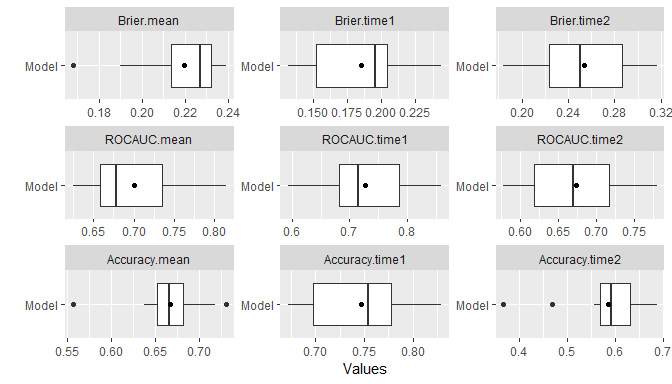The summary function when applied directly to output from resample computes default performance metrics as described in the Performance Function section. Likewise, the metricinfo and performance functions can be applied to the output in order to list and compute applicable metrics.

## Resample-specific metrics
metricinfo(res_probs) %>% names
#>   "accuracy"    "auc"         "brier"       "f_score"     "fnr"
#>   "fpr"         "kappa2"      "npv"         "ppv"         "pr_auc"
#>  "precision"   "recall"      "roc_auc"     "roc_index"   "rpp"
#>  "sensitivity" "specificity" "tnr"         "tpr"

## User-specified survival probability metrics
summary(performance(res_probs, metrics = c(sensitivity, specificity)))
#>                        Mean    Median         SD       Min       Max NA
#> sensitivity.mean  0.2433420 0.2455747 0.08327312 0.1309881 0.3992344  0
#> sensitivity.time1 0.2242094 0.2286585 0.13007156 0.0000000 0.5000000  0
#> sensitivity.time2 0.2624746 0.2404528 0.09444349 0.1262664 0.4489532  0
#> specificity.mean  0.9052404 0.9213255 0.06184367 0.7229870 0.9686275  0
#> specificity.time1 0.9423829 0.9372549 0.03467425 0.8660050 1.0000000  0
#> specificity.time2 0.8680980 0.8760562 0.09670937 0.5799691 1.0000000  0

## 7.4 Stratified Resampling

Stratification of cases for the construction of resampled training and test sets can be employed to help achieve balance across the sets. Stratified resampling is automatically performed if variable specification is in terms of a traditional formula and will be done according to the response variable if a numeric vector or factor, the event variable if survival, and the first variable if a numeric matrix. For model frames and recipes, stratification variables must be defined explicitly with the strata argument to the ModelFrame constructor or with the "case_strata" role designation in a recipe step.

## Model frame with case status stratification
mf <- ModelFrame(surv_fo, data = surv_df, strata = status)

resample(mf, model = GBMModel)

## Recipe with case status stratification
rec <- recipe(time + status ~ ., data = surv_df) %>%

resample(rec, model = GBMModel)

## 7.5 Resampling for Model Comparisons

Resampled metrics from different models can be combined for comparison with the Resamples function. Optional names given on the left hand side of equal operators within calls to Resamples will be used as labels in output from the summary and plot functions. For comparisons of resampled output, the same control structure must be used in all associated calls to resample to ensure that resulting model metrics are computed on the same resampled training and test sets.

## Resample estimation
res1 <- resample(surv_fo, data = surv_df, model = GBMModel(n.trees = 25),
control = surv_means_control)
res2 <- resample(surv_fo, data = surv_df, model = GBMModel(n.trees = 50),
control = surv_means_control)
res3 <- resample(surv_fo, data = surv_df, model = GBMModel(n.trees = 100),
control = surv_means_control)

## Combine resample output for comparison
(res <- Resamples(GBM1 = res1, GBM2 = res2, GBM3 = res3))
#> An object of class "Resamples"
#>
#> Models: GBM1, GBM2, GBM3
#> Stratification variable: (strata)
#>
#> An object from class "MLControl"
#>
#> Name: CVControl
#> Label: K-Fold Cross-Validation
#> Folds: 5
#> Repeats: 3
#> Seed: 123

summary(res)
#> , , CIndex
#>
#>           Mean    Median         SD       Min       Max NA
#> GBM1 0.7122178 0.7014388 0.06433288 0.6289238 0.8386243  0
#> GBM2 0.7058983 0.6883562 0.06256998 0.6300000 0.8359788  0
#> GBM3 0.6941198 0.6762295 0.05933365 0.6244541 0.8227513  0

plot(res)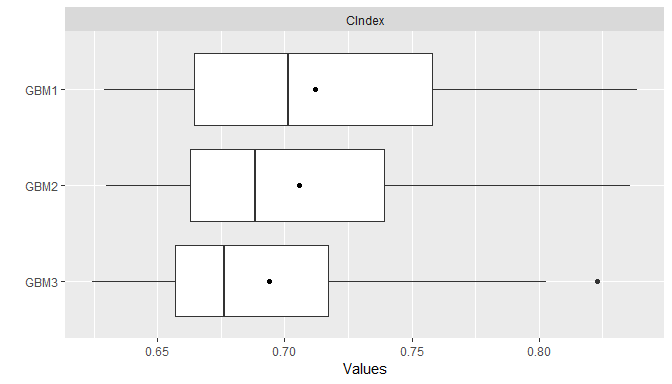plot(res, type = "density")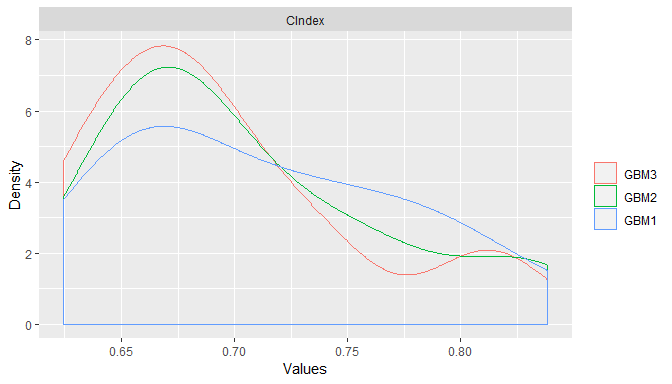plot(res, type = "errorbar")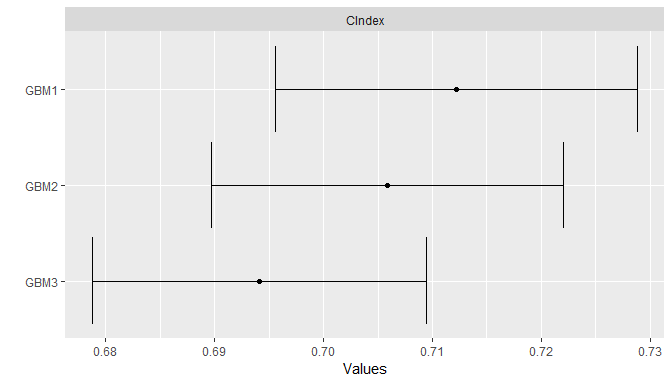plot(res, type = "violin")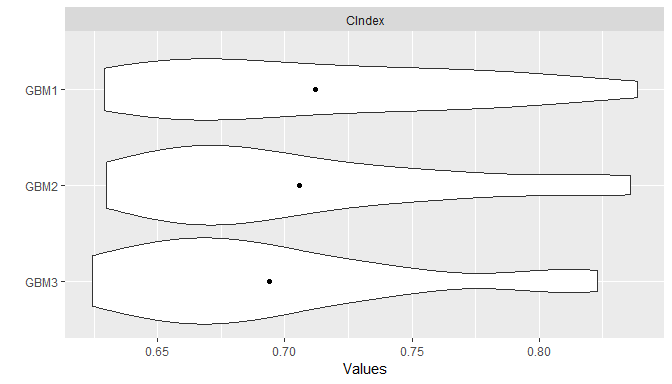Pairwise model differences for each metric can be calculated with the diff function applied to results from a call to Resamples. The differences can be summarized descriptively with the summary and plot functions and assessed for statistical significance with the t.test function.

## Pairwise model comparisons
(perfdiff <- diff(res))
#> An object of class "PerformanceDiff"
#>
#> Metrics: CIndex
#> Models: GBM1 - GBM2, GBM1 - GBM3, GBM2 - GBM3

summary(perfdiff)
#> , , CIndex
#>
#>                    Mean      Median         SD         Min        Max NA
#> GBM1 - GBM2 0.006319491 0.004366812 0.01613621 -0.02494062 0.03881279  0
#> GBM1 - GBM3 0.018098015 0.019464720 0.02692342 -0.03026906 0.05479452  0
#> GBM2 - GBM3 0.011778524 0.015267176 0.01695738 -0.01793722 0.04449153  0

plot(perfdiff)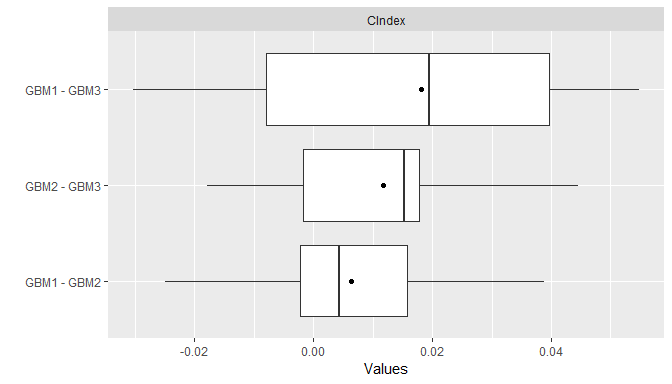t.test(perfdiff)
#> An object of class "HTestPerformanceDiff"
#>
#> Upper diagonal: mean differences (row - column)
#> Lower diagonal: p-values
#>
#> , , CIndex
#>
#>            GBM1        GBM2       GBM3
#> GBM1         NA 0.006319491 0.01809802
#> GBM2 0.15157114          NA 0.01177852
#> GBM3 0.05277722 0.052777218         NA

# 8 Graphical and Tabular Performance Summaries

## 8.1 Variable Importance

The importance of variables in a model fit is estimated with the varimp function and plotted with plot. Variable importance is a measure of the relative importance of predictors in a model and has a default range of 0 to 100, where 0 denotes the least important variables and 100 the most.

## Predictor variable importance
(vi <- varimp(surv_fit))
#>             Overall
#> thickness 100.00000
#> year       53.78039
#> age        50.98203
#> ulcer      20.00854
#> sex         0.00000

plot(vi)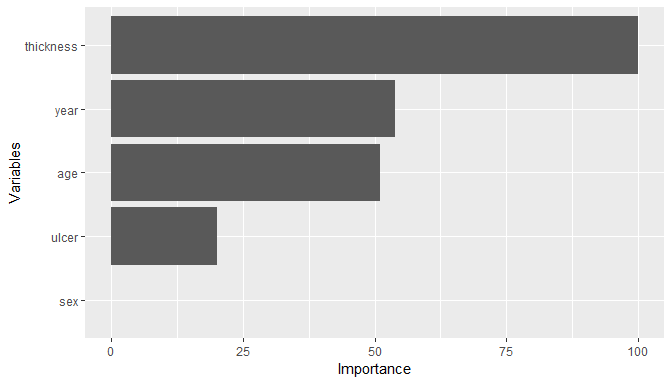## 8.2 Calibration Curves

Agreement between model-predicted and observed values can be visualized with calibration curves. In the construction of these curves, cases are partitioned into bins according to their (resampled) predicted responses. Mean observed responses are then calculated within each of the bins and plotted on the vertical axis against the bin midpoints on the horizontal axis. An option to produce curves smoothed over the individual predicted values is also provided. Calibration curves that are close to the 45-degree line indicate close agreement between observed and predicted responses and a model that is said to be well calibrated.

## Binned calibration curves
cal <- calibration(res_probs, breaks = 10)
plot(cal, se = TRUE)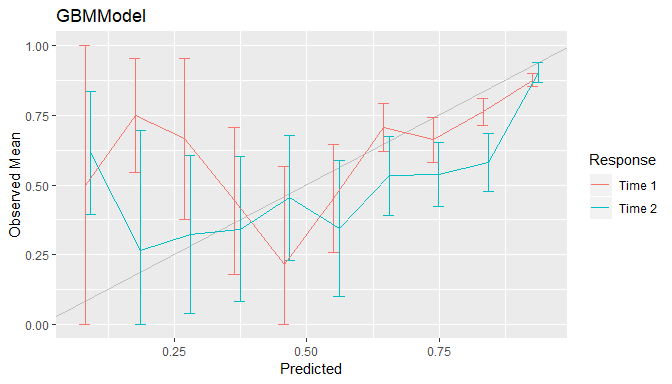## Smoothed calibration curves
cal <- calibration(res_probs, breaks = NULL)
plot(cal)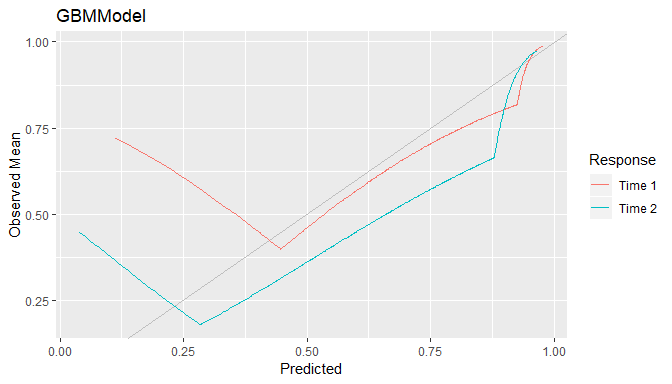## 8.3 Confusion Matrices

Confusion matrices of cross-classified observed and predicted factor responses are available with the confusion function. They can be constructed with predicted class membership or with predicted class probabilities. In the latter case, predicted class membership is derived from predicted probabilities according to a probability cutoff value for binary factors and according to the class with highest probability for factors with more than two levels. Performance metrics, such as those described earlier for binary factors, can be computed with the performance function and summarized with summary and plot.

## Confusion matrices
(conf <- confusion(res_probs, cutoff = 0.5))
#> GBMModel.time1 :
#>          Observed
#> Predicted         0         1
#>         0 424.44865 129.55135
#>         1  25.89286  35.10714
#>
#> GBMModel.time2 :
#>          Observed
#> Predicted         0         1
#>         0 297.18631 201.81369
#>         1  44.34498  71.65502

performance(conf, metrics = c("Accuracy" = accuracy,
"Sensitivity" = sensitivity,
"Specificity" = specificity))
#> GBMModel.time1 :
#>    Accuracy Sensitivity Specificity
#>   0.7472452   0.2132119   0.9425040
#>
#> GBMModel.time2 :
#>    Accuracy Sensitivity Specificity
#>   0.5997420   0.2620227   0.8701584

summary(conf)
#> GBMModel.time1 :
#> Number of responses: 615
#> Accuracy (SE): 0.7472452 (0.01752442)
#> Majority class: 0.7322626
#> Kappa: 0.1945668
#>
#>                     0          1
#> Observed    0.7322626 0.26773739
#> Predicted   0.9008130 0.09918699
#> Agreement   0.6901604 0.05708479
#> Sensitivity 0.9425040 0.21321185
#> Specificity 0.2132119 0.94250395
#> PPV         0.7661528 0.57552693
#> NPV         0.5755269 0.76615280
#>
#> GBMModel.time2 :
#> Number of responses: 615
#> Accuracy (SE): 0.599742 (0.01975671)
#> Majority class: 0.5553354
#> Kappa: 0.1402268
#>
#>                     0         1
#> Observed    0.5553354 0.4446646
#> Predicted   0.8113821 0.1886179
#> Agreement   0.4832298 0.1165122
#> Sensitivity 0.8701584 0.2620227
#> Specificity 0.2620227 0.8701584
#> PPV         0.5955637 0.6177157
#> NPV         0.6177157 0.5955637
plot(conf)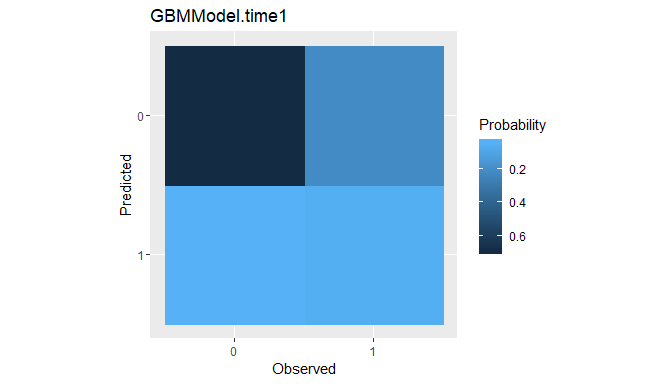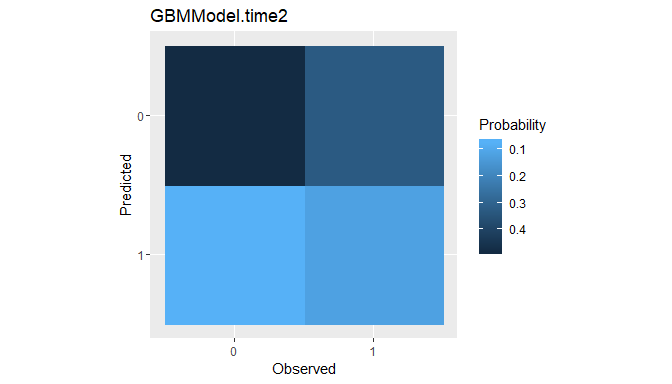## 8.4 Partial Dependence Plots

Partial dependence plots display the marginal effects of predictors on the response variable. The response scale displayed in the plots will depend on the response type: probability for factors and predicted survival probabilities, original scale for numerics, and survival time for predicted survival means.

## Partial dependence plots
pd <- dependence(surv_fit, select = c(thickness, age))
plot(pd)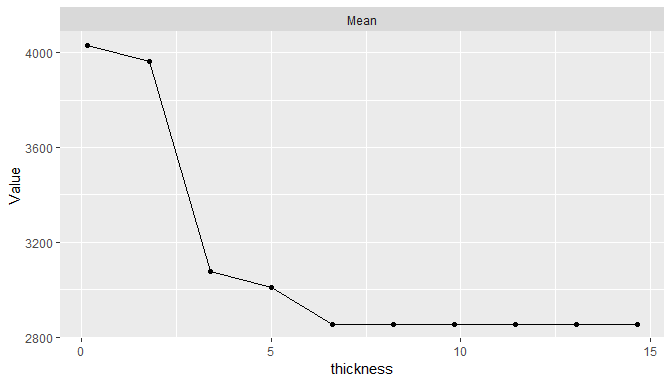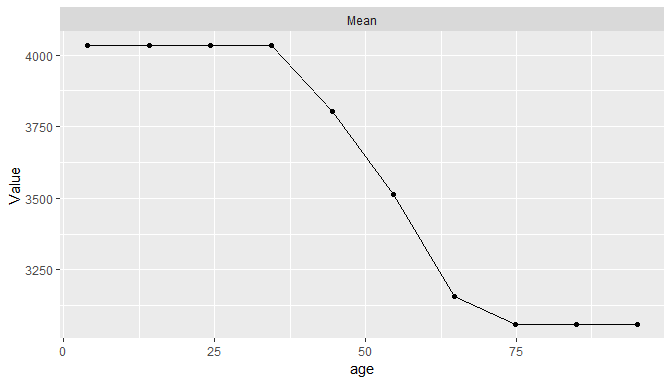## 8.5 Performance Curve Analysis

Tradeoffs between correct and incorrect classifications of binary outcomes, across the range of possible cutoff probabilities, can be studied with performance curves.

### 8.5.1 ROC Curves

Receiver operating characteristic (ROC) curves are one example in which true positive rates (sensitivity) are plotted against false positive rates (1 - specificity). Area under resulting ROC curves can be computed as an overall measure of model predictive performance and interpreted as the probability that a randomly selected event case will have a higher predicted value than a randomly selected non-event case.

## ROC curves
roc <- performance_curve(res_probs)
plot(roc, diagonal = TRUE)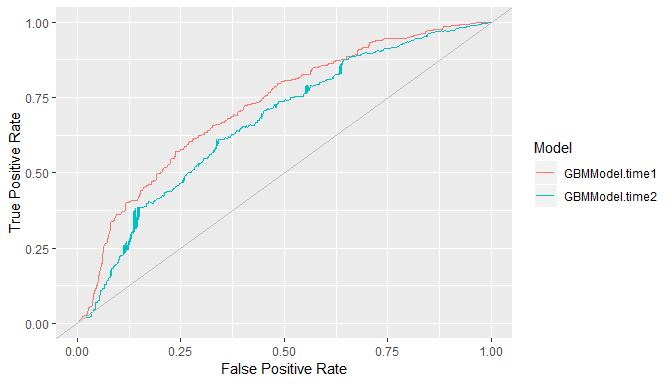plot(roc, type = "cutoffs")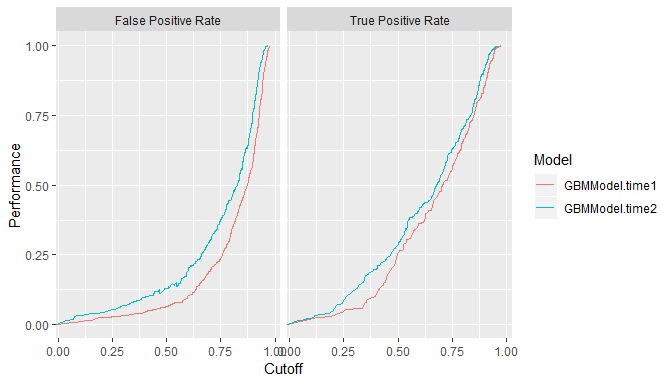auc(roc)
#> Model: GBMModel.time1
#>  0.7209396
#> --------------------------------------------------------
#> Model: GBMModel.time2
#>  0.6683252

### 8.5.2 Precision Recall Curves

In general, any two binary response metrics may be specified for the construction of a performance curve. Precision recall curves are another example.

## Precision recall curves
pr <- performance_curve(res_probs, metrics = c(precision, recall))
plot(pr)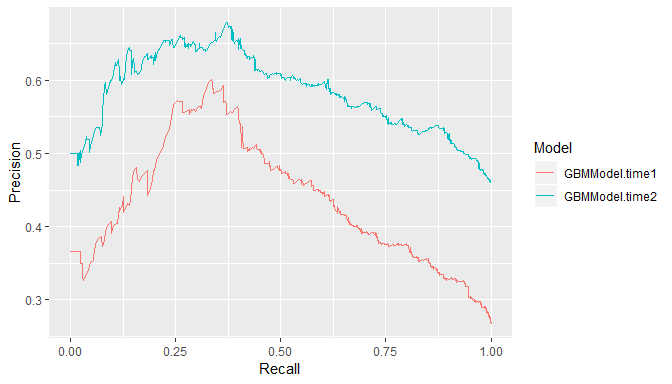auc(pr)
#> Model: GBMModel.time1
#>  0.4343253
#> --------------------------------------------------------
#> Model: GBMModel.time2
#>  0.5826389

### 8.5.3 Lift Curves

Lift curves depict the rate at which observed binary responses are identifiable from (resampled) predicted response probabilities. In particular, they plot the true positive findings (sensitivity) against the positive test rates for all possible classification probability cutoffs. Accordingly, a lift curve can be interpreted as the rate at which positive responses are found as a function of the positive test rate among cases.

## Lift curves
lf <- lift(res_probs)
plot(lf, find = 0.75)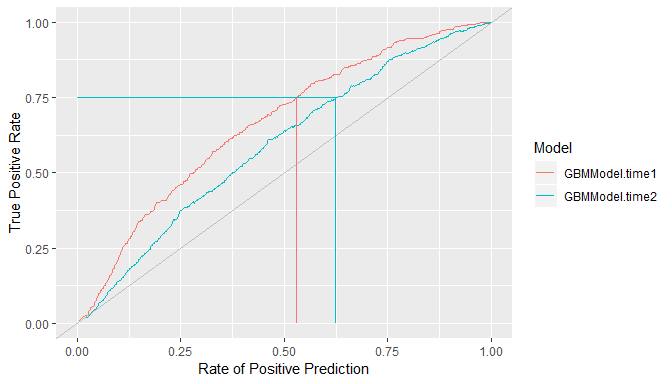# 9 Modeling Strategies

## 9.1 Model Tuning

Many of the modeling functions have arguments, or parameters, that control aspects of their model fitting algorithms. For example, GBMModel parameters n.trees and interaction.depth control the number of decision trees to fit and the maximum depth of variable interactions. The tune function performs model fitting over a grid of parameter values and returns the model with the most optimal values. Optimality is determined based on the first performance metric supplied to the metrics argument of tune. Furthermore, argument grid controls the construction of grid values and can be a single numeric value giving the grid length in each parameter dimension, a call to Grid with the grid length and number of grid points to sample at random, or a user-specified data frame of grid points. Summary statistics and plots of resulting performances across all metrics and tuning parameters can be obtained with the summary and plot functions.

## Tune over automatic grid of model parameters
(surv_tune <- tune(surv_fo, data = surv_df, model = GBMModel,
grid = 3,
control = surv_means_control,
metrics = c("CIndex" = cindex, "RMSE" = rmse)))
#> An object of class "MLModelTune"
#>
#> Model name: GBMModel
#> Label: Generalized Boosted Regression
#> Packages: gbm
#> Response types: factor, numeric, Surv
#>
#> Parameters:
#> $n.trees #>  50 #> #>$interaction.depth
#>  1
#>
#> $n.minobsinnode #>  10 #> #>$shrinkage
#>  0.1
#>
#> \$bag.fraction
#>  0.5
#>
#> Grid:
#>   n.trees interaction.depth
#> 1      50                 1
#> 2     100                 1
#> 3     150                 1
#> 4      50                 2
#> 5     100                 2
#> 6     150                 2
#> 7      50                 3
#> 8     100                 3
#> 9     150                 3
#>
#> An object of class "Performance"
#>
#> Metrics: CIndex, RMSE
#> Models: GBMModel.1, GBMModel.2, GBMModel.3, GBMModel.4, GBMModel.5, GBMModel.6, GBMModel.7, GBMModel.8, GBMModel.9
#>
#> Selected (CIndex): GBMModel.1

summary(surv_tune)
#> , , CIndex
#>
#>                 Mean    Median         SD       Min       Max NA
#> GBMModel.1 0.7058983 0.6883562 0.06256998 0.6300000 0.8359788  0
#> GBMModel.2 0.6941198 0.6762295 0.05933365 0.6244541 0.8227513  0
#> GBMModel.3 0.6850918 0.6745843 0.06864062 0.5805085 0.8174603  0
#> GBMModel.4 0.6961474 0.6886792 0.05489630 0.6207627 0.8028504  0
#> GBMModel.5 0.6828937 0.6792453 0.06191098 0.5677966 0.8015873  0
#> GBMModel.6 0.6785267 0.6933962 0.06486174 0.5868644 0.7885986  0
#> GBMModel.7 0.6925677 0.6721311 0.05600214 0.6165254 0.8004751  0
#> GBMModel.8 0.6778869 0.6650943 0.05688398 0.5889831 0.7838480  0
#> GBMModel.9 0.6709213 0.6745283 0.06440546 0.5805085 0.8028504  0
#>
#> , , RMSE
#>
#>                Mean   Median       SD      Min      Max NA
#> GBMModel.1 2641.262 2647.588 329.4775 2015.310 3229.041  0
#> GBMModel.2 2729.431 2716.819 351.6683 2099.813 3260.165  0
#> GBMModel.3 2762.201 2721.302 388.5256 2059.193 3346.956  0
#> GBMModel.4 2739.729 2737.601 356.4921 2010.709 3245.199  0
#> GBMModel.5 2862.102 2903.612 391.1429 2132.708 3533.432  0
#> GBMModel.6 2933.915 2954.276 392.4251 2096.266 3482.183  0
#> GBMModel.7 2803.999 2830.390 350.3109 2026.888 3290.263  0
#> GBMModel.8 2976.085 3023.242 382.5607 2184.120 3517.882  0
#> GBMModel.9 3075.965 3143.521 413.9979 2161.720 3668.848  0

plot(surv_tune, type = "line")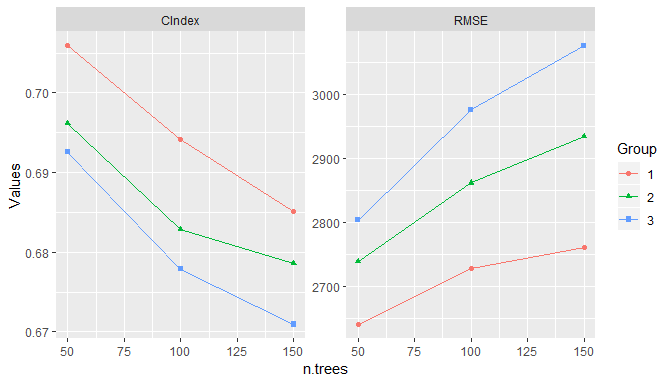## Tune over randomly sampled grid points
tune(surv_fo, data = surv_df, model = GBMModel,
grid = Grid(length = 100, random = 10),
control = surv_means_control)

## Tune over user-specified grid points
tune(surv_fo, data = surv_df, model = GBMModel,
grid = expand.grid(n.trees = c(25, 50, 100),
interaction.depth = 1:3),
control = surv_means_control)

The return value of tune is a model object with the optimal tuning parameters and not a model fit object. The returned model can be fit subsequently to a set of data with the fit function.

## Fit the tuned model
surv_fit <- fit(surv_fo, data = surv_df, model = surv_tune)
(vi <- varimp(surv_fit))
#>             Overall
#> thickness 100.00000
#> age        66.54478
#> ulcer      34.04836
#> year       12.62582
#> sex         0.00000

## 9.2 Model Selection

Model selection can be performed with the tune function to select from any combination of models and model parameters. It has as a special case the just-discussed tuning of a single model over a grid of parameter values. In general, a list containing any combination of model functions, function names, and function calls can be supplied to the models argument of tune to perform model selection. An expand.model helper function is additionally provided to expand a model over a grid of tuning parameters for inclusion in the list if so desired. In this general form of model selection, the grid argument discussed previously for grid tuning is not used.

## Select from a list of candidate models
model_list <- c(
expand.model(GBMModel, n.trees = c(50, 100), interaction.depth = 1:2),
GLMNetModel(lambda = 0.01),
CoxModel,
SurvRegModel
)

tune(surv_fo, data = surv_df, models = model_list,
control = surv_means_control)

## 9.3 Ensemble Models

Ensemble methods combine multiple base learning algorithms as a strategy to improve predictive performance. Two ensemble methods implemented in Machineshop are stacked regression (Breiman 1996) and super learners (Lann and Hubbard 2007). Stacked regression fits a linear combination of resampled predictions from specified base learners; whereas, super learners fit a specified model, such as GBMModel, to the base learner predictions and optionally also to the original predictor variables. Illustrated below is a performance evaluation of stacked regression and a super learner fit to gradient boosted, random forest, and Cox regression base learners. In the second case, a separate gradient boosted model is used as the super learner.

## Stacked regression
stackedmodel <- StackedModel(GLMBoostModel, CForestModel, CoxModel)
res_stacked <- resample(surv_fo, data = surv_df, model = stackedmodel)
summary(res_stacked)
#>             Mean    Median        SD  Min       Max NA
#> CIndex 0.6960906 0.7205175 0.1134739 0.48 0.8378378  0

## Super learner
supermodel <- SuperModel(GLMBoostModel, CForestModel, CoxModel,
model = GBMModel)
res_super <- resample(surv_fo, data = surv_df, model = supermodel)
summary(res_super)
#>            Mean    Median        SD      Min   Max NA
#> CIndex 0.707372 0.6994528 0.1068744 0.468254 0.845  0

# 10 User-Defined Models and Metrics

Custom models and metrics can be defined with the MLModel and MLMetric constructors for use with the model fitting, prediction, and performance assessment tools provided by the package.

## Logistic regression model
LogisticModel <- MLModel(
name = "LogisticModel",
types = "binary",
fit = function(formula, data, weights, ...) {
glm(formula, data = data, weights = weights, family = binomial, ...)
},
predict = function(object, newdata, ...) {
predict(object, newdata = newdata, type = "response")
},
varimp = function(object, ...) {
pchisq(coef(object)^2 / diag(vcov(object)), 1)
}
)

## F2 score metric
f2_score <- MLMetric(
function(observed, predicted, ...) {
f_score(observed, predicted, beta = 2, ...)
},
name = "f2_score",
label = "F2 Score",
maximize = TRUE
)

library(MASS)
res <- resample(type ~ ., data = Pima.tr, model = LogisticModel)
summary(performance(res, metric = f2_score))
#>               Mean    Median        SD       Min       Max NA
#> f2_score 0.5697769 0.6060606 0.1924873 0.1666667 0.8571429  0

# 11 Appendix

## 11.1 Model Constructor Functions

Table A1. Package-supplied model constructor functions and supported response variable types.
Response Variable Types
Function Label Categorical1 Continuous2 Survival3
AdaBagModel Bagging with Classification Trees f
AdaBoostModel Boosting with Classification Trees f
BARTModel Bayesian Additive Regression Trees f n S
BARTMachineModel Bayesian Additive Regression Trees b n
BlackBoostModel Gradient Boosting with Regression Trees b n S
C50Model C5.0 Classification f
CForestModel Conditional Random Forests f n S
CoxModel Cox Regression S
CoxStepAICModel Cox Regression (Stepwise) S
EarthModel Multivariate Adaptive Regression Splines f n
FDAModel Flexible Discriminant Analysis f
GBMModel Generalized Boosted Regression f n S
GLMBoostModel Gradient Boosting with Linear Models b n S
GLMModel Generalized Linear Models b n
GLMStepAICModel Generalized Linear Models (Stepwise) b n
GLMNetModel Lasso and Elastic-Net f m, n S
KNNModel K-Nearest Neighbors Model f, o n
LARSModel Least Angle Regression n
LDAModel Linear Discriminant Analysis f
LMModel Linear Model f m, n
MDAModel Mixture Discriminant Analysis f
NaiveBayesModel Naive Bayes Classifier f
NNetModel Feed-Forward Neural Networks f n
PDAModel Penalized Discriminant Analysis f
PLSModel Partial Least Squares f n
POLRModel Ordered Logistic Regression o
RandomForestModel Random Forests f n
RangerModel Fast Random Forests f n S
RPartModel Recursive Partitioning and Regression Trees f n S
StackedModel Stacked Regression f, o m, n S
SuperModel Super Learner f, o m, n S
SurvRegModel Parametric Survival S
SurvRegStepAICModel Parametric Survival (Stepwise) S
SVMModel Support Vector Machines f n
SVMANOVAModel Support Vector Machines (ANOVA) f n
SVMBesselModel Support Vector Machines (Bessel) f n
SVMLaplaceModel Support Vector Machines (Laplace) f n
SVMLinearModel Support Vector Machines (Linear) f n
SVMPolyModel Support Vector Machines (Poly) f n
SVMSplineModel Support Vector Machines (Spline) f n
SVMTanhModel Support Vector Machines (Tanh) f n
TreeModel Regression and Classification Trees f n
XGBModel Extreme Gradient Boosting f n
XGBDARTModel Extreme Gradient Boosting (DART) f n
XGBLinearModel Extreme Gradient Boosting (Linear) f n
XGBTreeModel Extreme Gradient Boosting (Tree) f n
1 b = binary factor, f = factor, o = ordered factor
2 m = matrix, n = numeric
3 S = Surv

## 11.2 Metric Functions

Table A2. Package-supplied performance metric functions and supported response variable types.
Response Variable Types
Function Label Categorical1 Continuous2 Survival3
accuracy Accuracy f S
auc Area Under Performance Curve b S
brier Brier Score f S
cindex Concordance Index b S
cross_entropy Cross Entropy f
f_score F Score b S
fnr False Negative Rate b S
fpr False Positive Rate b S
gini Gini Coefficient m, n S
kappa2 Cohen’s Kappa f S
mae Mean Absolute Error m, n S
mse Mean Squared Error m, n S
msle Mean Squared Log Error m, n S
npv Negative Predictive Value b S
ppv Positive Predictive Value b S
pr_auc Area Under Precision-Recall Curve b S
precision Precision b S
r2 Coefficient of Determination m, n S
recall Recall b S
rmse Root Mean Squared Error m, n S
rmsle Root Mean Squared Log Error m, n S
roc_auc Area Under ROC Curve b S
roc_index ROC Index b S
rpp Rate of Positive Prediction b S
sensitivity Sensitivity b S
specificity Specificity b S
tnr True Negative Rate b S
tpr True Positive Rate b S
weighted_kappa2 Weighted Cohen’s Kappa o
1 b = binary factor, f = factor, o = ordered factor
2 m = matrix, n = numeric
3 S = Surv